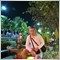• 概要
• レビュー (1)
• コメント

# Smart Ruler MT5

This is a convenient tool for measuring the number of points between prices. It support magnetizing to OHLC prices. Calculates profit considering specified lot size and spread (optionally). It counts the number of bars between specified point and the time difference between them. Calculates the slope angle from the horizontal position. Unneeded parameters can be hidden. It does not occupy much space when inactive.

Once you add the indicator on the chart, Ruler caption will appear (according to specified X distance, Y distance). In order to activate the measure mode, click it with the mouse. A table with parameters will appear. In order to measure, set coordinates for two points on the chart using the mouse. As soon as you finish measuring, click on Ruler again in order to deactivate the measure mode.

### Input Parameters

• X distance - X coordinate for "Ruler" caption.
• Y distance - Y coordinate for "Ruler" caption.
• Magnet area - magnet sensitivity in points.
• Display points - display distance (absolute value) between prices in points (see Note 1).
• Display profit - display calculated profit value in base currency.
• Consider the spread - consider current spread value when calculating the profit.
• Lot size - lot size to calculate the profit.
• Display bars count - display the number of bars between specified points considering outside left and right bars (if the start and end match, value 1 is displayed).
• Display time diff - display time difference between the specified points.
• Display angle - display the absolute value of the horizontal deviation of the line drawn (see. Note 2), disabled on default.
• Angle coeff - coefficient for the time scale used for calculation of the slope angle.
• Text color (active) - text color (measure mode active).
• Text color (inactive) - text color (measure mode inactive).
• Line color - line color.
• Display projections - display projections.

Note 1: the distance between two prices in point is forced to be displayed in case no parameters are selected.

Note 2: the horizontal deviation is more a symbolic value. It is calculated as a = arctan(dpp/(k*len)), where dpp is the distance in points, len is the number of bars (including outside bars), k is the coefficient of the time scale (parameter Angle coeff). The coefficient is chosen for every price and time scale individually.1008
2015.05.09 19:34

Полезный инструмент!

Советую попробовать

Спасибо Автору